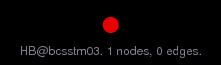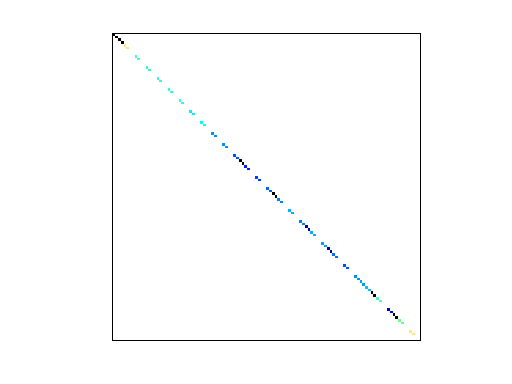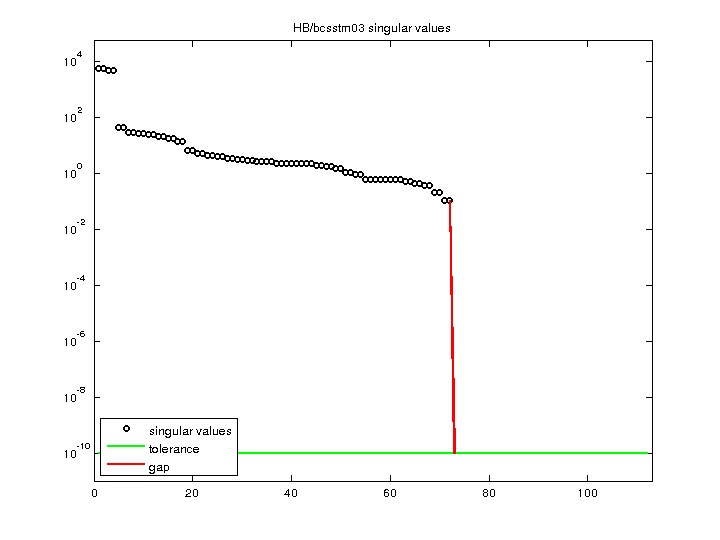Matrix: HB/bcsstm03

Description: SYMMETRIC MASS MATRIX, SMALL TEST STRUCTURE(undirected graph drawing)• Matrix group: HB
• download as a MATLAB mat-file, file size: 1 KB. Use UFget(58) or UFget('HB/bcsstm03') in MATLAB.

 Matrix properties number of rows 112 number of columns 112 nonzeros 72 structural full rank? no structural rank 72 # of blocks from dmperm 74 # strongly connected comp. 112 explicit zero entries 40 nonzero pattern symmetry symmetric numeric value symmetry symmetric type real structure symmetric Cholesky candidate? no positive definite? no

 author J. Lewis editor I. Duff, R. Grimes, J. Lewis date 1982 kind structural problem 2D/3D problem? yes

 Ordering statistics: result nnz(chol(P*(A+A'+s*I)*P')) with AMD 112 Cholesky flop count 1.1e+02 nnz(L+U), no partial pivoting, with AMD 112 nnz(V) for QR, upper bound nnz(L) for LU, with COLAMD 112 nnz(R) for QR, upper bound nnz(U) for LU, with COLAMD 112

Note that all matrix statistics (except nonzero pattern symmetry) exclude the 40 explicit zero entries.

 SVD-based statistics: norm(A) 5403.5 min(svd(A)) 0 cond(A) Inf rank(A) 72 sprank(A)-rank(A) 0 null space dimension 40 full numerical rank? no singular value gap Inf

 singular values (MAT file): click here SVD method used: s = svd (full (A)) ; status: ok